Engineering Jobs   »   Mechincal Engineering quizs   »   UPPSC Lecturer Recruitment 2021, UPPSC Lecturer...

# UPPSC Lec’21 ME: Daily Practices Quiz. 02-Dec-2021

Quiz: Mechanical Engineering
Exam: UPPSC AE/Lecturer
Topic: Miscellaneous

Each question carries 3 marks
Negative marking: 1 mark
Time: 8 Minutes

Q1. Two large parallel grey plates with a small gap, exchange radiation at the rate of 1000 W/m2 when their emissivity are 0.5 each. By coating one plate, its emissivity is reduced to 0.25. Temperatures remain unchanged. The new rate of heat exchange shall become
(a) 500 W/m2
(b) 600 W/m2
(C) 700 W/m2
(d) 800 W/m2

Q2. Two long parallel plates of same emissivity 0.5 are maintained at different temperatures and have radiation heat exchange between them. The radiation shield of emissivity 0.25 placed in the middle will reduce radiation heat exchange to
(a) 1/2
(b) 1/4
(c) 3/10
(d) 3/5

Q3. For a surface, the irradiation, radiosity and emissive power are 20, 12 and 10 W/m2 respectively. The emissivity of the surface is?
(a) 0.2
(b) 0.4
(c) 0.9
(d) 1.0

Q4. In PERT and CPM network the dummy activity
(a) consumes time
(b) consumes resources
(c) is used to preserve the logic
(d) is a real activity

Q5. Stirling cycle consists of
(a) Two constant volume & two isothermal processes
(b) Two isothermal and two isentropic processes
(c) Two constant volume and two isentropic processes
(d) One constant volume, one constant pressure and two isentropic processes

Q6. Perpetual motion machine of second kind (PMM-II) violates the
(a) Zeroth law of thermodynamics
(b) First law of thermodynamics
(c) Second law of thermodynamics
(d) Third law of thermodynamics
L1Difficulty 3
QTags Mechanical
QCreator Paper Maker 10

Solutions

S1. Ans.(b)
Sol.

S2. Ans.(c)
Sol.

Without shield, the heat transfer is Q_1
So,
Q_1=σ(T_1^4-T_2^4 )/(1/ε_1 +1/ε_2 -1)=σ(T_1^4-T_2^4 )/(1/0.5+1/0.5-1)
Q_1=σ(T_1^4-T_2^4 )/3
With shield the heat transfer is Q_2
Q_2=σ(T_1^4-T_2^4 )/(1/ε_1 +1/ε_2 +2/ε_S -2)
Q_2=σ(T_1^4-T_2^4 )/(1/0.5+1/0.5+2/0.25-2)=σ(T_1^4-T_2^4 )/10
So,
Q_2/Q_1 =3/10
∴Q_2=3/10 Q_1

S3. Ans.(c)
Sol. Given that,
Radiosity (J) = 12 Irradiation = 20 and Emissive power = 10
We know that,
J = E_b + (1 – )G
12 = (10) + (1 – )20
12 = 10 + 20 – 20
18 = 20
ε=18/20=0.9

S4. Ans.(c)
Sol. In PERT and CPM network the dummy activity is used to preserve the logic

S5. Ans. (b)
Sol. Stirling cycle consists of two constant volume and two isothermal processes.

S6. Ans. (c)
Sol. Perpetual motion machine of second kind (PMM-II) violates the second law of thermodynamics.

Sharing is caring!

•GATE 2023 Admit Card Out, Direct Link fo...
•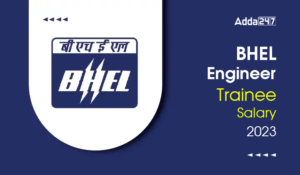BHEL Engineer Trainee Salary 2023, Perks...
•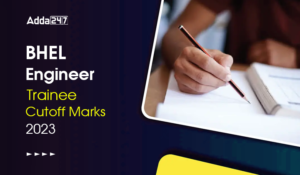BHEL Engineer Trainee Cutoff Marks 2023,...
•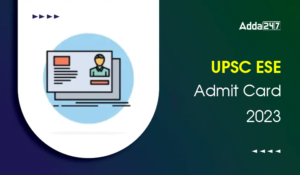UPSC ESE Admit Card 2023, Download Preli...
•Last Week Preparation Strategy for GATE ...
•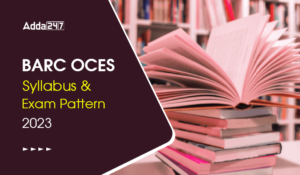BARC OCES Syllabus 2023 Checkout Latest ...
•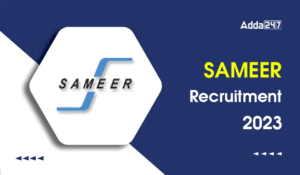SAMEER Recruitment 2023 Out For 35 Posts...